информационная безопасность
без паники и всерьезподробно о проекте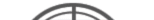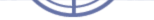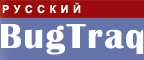Анализ криптографических сетевых...Модель надежности двухузлового...Специальные марковские модели надежности...Phrack #70/0x46Возможно, Facebook наступил на...50 лет электронной почте
 главная обзор RSN блог библиотека закон бред форум dnet о проектеbugtraq.ru / форум / programming
 Имя ПарольФОРУМвсе доски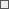FAQIRCновые сообщенияsite updatesguestbookbeginnerssysadminprogramming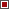operating systemstheoryweb buildingsoftwarehardwarenetworkinglawhackinggadgetsjobdnethumormiscellaneousscrapрегистрацияЛегенда: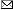новое сообщение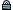закрытая нитка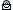новое сообщениев закрытой нитке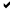старое сообщение• Напоминаю, что масса вопросов по функционированию форума снимается после прочтения его описания.
• Новичкам также крайне полезно ознакомиться с данным документом.
[C++] Ну вот, все видно 03.03.12 19:01  Число просмотров: 2028
Автор: leo <Леонид Юрьев> Статус: Elderman
Отредактировано 03.03.12 19:01  Количество правок: 1
```#include <stdio.h>

void x()
{
long long l64 = -2;
long long res = l64 / sizeof(short);

printf("x) arch=%d.bit, sizeof(long long) = %d, res = %lld = 0x%llX\n", (unsigned)sizeof(void*) * 8, (unsigned)sizeof(long long), res, res);
}

void y()
{
long long l64 = -2;
long long res = l64 / ( (unsigned long)sizeof(short) );

printf("y) arch=%d.bit, sizeof(long long) = %d, res = %lld = 0x%llX\n", (unsigned)sizeof(void*) * 8, (unsigned)sizeof(long long), res, res);
}

void z()
{
long long l64 = -2;
long long res = ( (unsigned long long)l64 ) / sizeof(short);

printf("z) arch=%d.bit, sizeof(long long) = %d, res = %lld = 0x%llX\n", (unsigned)sizeof(void*) * 8, (unsigned)sizeof(long long), res, res);
}

int main(int argc, char** argv)
{
x();
y();
z();
}
```

---

gcc -m32 x.c && ./a.out
x) arch=32.bit, sizeof(long long) = 8, res = -1 = 0xFFFFFFFFFFFFFFFF
y) arch=32.bit, sizeof(long long) = 8, res = -1 = 0xFFFFFFFFFFFFFFFF
z) arch=32.bit, sizeof(long long) = 8, res = 9223372036854775807 = 0x7FFFFFFFFFFFFFFF

gcc -m64 x.c && ./a.out
x) arch=64.bit, sizeof(long long) = 8, res = 9223372036854775807 = 0x7FFFFFFFFFFFFFFF
y) arch=64.bit, sizeof(long long) = 8, res = 9223372036854775807 = 0x7FFFFFFFFFFFFFFF
z) arch=64.bit, sizeof(long long) = 8, res = 9223372036854775807 = 0x7FFFFFFFFFFFFFFFПоиск
 [C++] на 64bit компайлере вот такая поганка - + 28.02.12 01:23  Могу ошибаться, но, вроде бы как в стандарте не ст... (-)  - kstati 07.03.12 08:18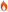sizeof() - size_t - разный размер в зависимости от платформы (-)  - :-) 07.03.12 18:08  BTW, а какой компилятор? (-)  - AlexD 02.03.12 14:50  на 2-х разных пробовал и подозреваю что так на всех (-)  - + 02.03.12 20:31  это были OpenSource'ные компиляторы? Возможно они... (-)  - Den 02.03.12 21:08  майкрософтовский и солярис (-)  - + 02.03.12 23:12  попробуй прописать директиву для 64-х битной модели,... - Den 03.03.12 04:29  похоже на ошибку оптимизации с тупым сдвигом регис... (-)  - Den 29.02.12 01:50  оптимизация отключена, debug build, - + 29.02.12 01:59  Почти RTFM - leo 28.02.12 19:53  ты внимательней прочти, а потом РТФэкай - + 29.02.12 01:28  может проблема в неявном приобразовании? - Den 29.02.12 13:31  почему 32 битный компайлер генерит другой код (правильный)? (-)  - + 01.03.12 07:48  Что-то не пойму я тебя - leo 29.02.12 12:20  а ну объясни, почему 32 битный компайлер генерит д... - + 01.03.12 07:47  [C++] Ну вот, все видно - leo 03.03.12 19:01  Короче, не нужно путать компилятор и он вас путать... (-)  - leo 03.03.12 19:03  ошибка разработчиков компилятора? (-)  - Den 01.03.12 11:08  проверил на другом компиляторе тоже самое (-)  - + 01.03.12 23:45